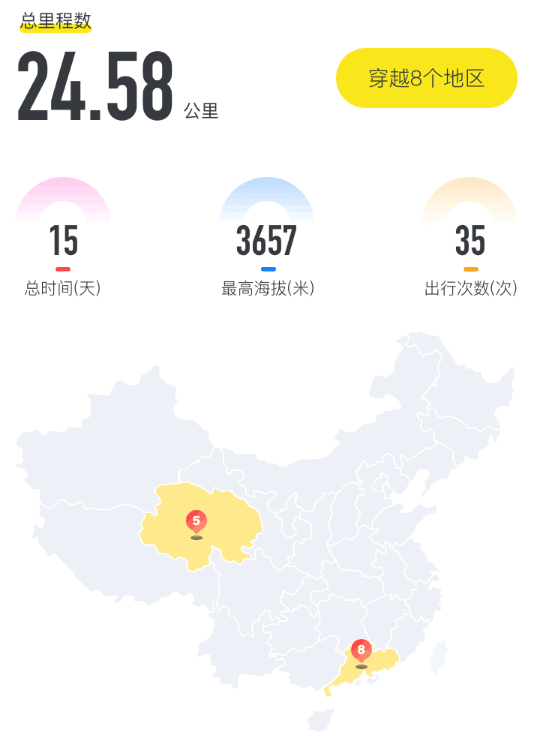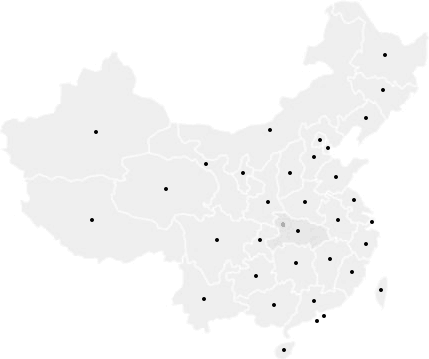# Android 绘制中国地图

2020/01/21 22:501. 中国地图
2. 高亮省区
3. 中心显示数字

## SVG Path

SVG：可缩放矢量图形（英语：Scalable Vector Graphics，SVG）是一种基于可扩展标记语言（XML），用于描述二维矢量图形的图形格式。元素是 SVG 基本形状中最强大的一个，它不仅能创建其他基本形状，还能创建更多其他形状。

### SVG Path 用 Android 绘制

 123 

 123456789101112131415161718192021222324252627282930  * 计算地图边界 * 1.黑龙江是中国最东，最北的省份 * 2.新疆是中国最西的省份 * 3.海南是中国最南的省份 */private fun () { val hljRF = RectF() xPaths[HEILONGJIANG_CODE]?.computeBounds(hljRF, true) val hnRF = RectF() xPaths[HAINAN_CODE]?.computeBounds(hnRF, true) mapWidth = hljRF.right mapHeight = hnRF.bottom}override fun onMeasure(widthMeasureSpec: Int, heightMeasureSpec: Int) { super.onMeasure(widthMeasureSpec, heightMeasureSpec) val speSize = View.MeasureSpec.getSize(widthMeasureSpec) scale = speSize / mapWidth setMeasuredDimension(speSize, (speSize * mapHeight / mapWidth).toInt())}override fun onDraw(canvas: Canvas) { super.onDraw(canvas) // 缩放画布 canvas.scale(scale, scale) ...}

M x,y 移动指令，映射 Path 中的 moveTo
L x,y 画直线指令，映射 Path 中的 lineTo
H x 画水平线指令，映射 Path 中的 lineTo，不过要使用上一个坐标的 y
V y 画垂直线指令，映射 Path 中的 lineTo，不过要使用上一个坐标的 x
C x1,y1,x2,y2,x,y 三次贝塞尔曲线指令，映射 Path 中的 cubicTo
S x2,y2,x,y 跟在 C 指令后面使用，用 C 指令的结束点做控制点，映射 cubicTo
T x,y 跟在 Q 指令后面使用，使用 Q 的 x,y 做控制点，映射 quadTo
Z path 关闭指令，映射 close

 12 M421.139,189.75L420.782,186.894M421.139,189.75l-0.357,-2.856

 123456789101112131415161718192021222324252627282930313233343536373839404142434445464748495051525354555657585960616263646566676869707172737475767778798081828384858687888990919293949596大专栏  Android 绘制中国地图"line">979899100101102103104105  * 仅限大写指令转换 */public class SvgPathToAndroidPath { private int svgPathLenght = 0; private String svgPath = null; private int mIndex; private List cmdPositions = new ArrayList<>(); * M x,y * L x,y * H x * V y * C x1,y1,x2,y2,x,y * Q x1,y1,x,y * S x2,y2,x,y * T x,y * */ public Path parser(String svgPath) { this.svgPath = svgPath; svgPathLenght = svgPath.length(); mIndex = 0; Path lPath = new Path(); lPath.setFillType(Path.FillType.WINDING); //记录最后一个操作点 PointF lastPoint = new PointF(); findCommand(); for (int i = 0; i < cmdPositions.size(); i++) { Integer index = cmdPositions.get(i); switch (svgPath.charAt(index)) { case 'M': { String ps[] = findPoints(i); lastPoint.set(Float.parseFloat(ps), Float.parseFloat(ps)); lPath.moveTo(lastPoint.x, lastPoint.y); } break; case 'L': { String ps[] = findPoints(i); lastPoint.set(Float.parseFloat(ps), Float.parseFloat(ps)); lPath.lineTo(lastPoint.x, lastPoint.y); } break; case 'H': {//基于上个坐标在水平方向上划线，因此y轴不变 String ps[] = findPoints(i); lastPoint.set(Float.parseFloat(ps), lastPoint.y); lPath.lineTo(lastPoint.x, lastPoint.y); } break; case 'V': {//基于上个坐标在水平方向上划线，因此x轴不变 String ps[] = findPoints(i); lastPoint.set(lastPoint.x, Float.parseFloat(ps)); lPath.lineTo(lastPoint.x, lastPoint.y); } break; case 'C': {//3次贝塞尔曲线 String ps[] = findPoints(i); lastPoint.set(Float.parseFloat(ps), Float.parseFloat(ps)); lPath.cubicTo(Float.parseFloat(ps), Float.parseFloat(ps), Float.parseFloat(ps), Float.parseFloat(ps), Float.parseFloat(ps), Float.parseFloat(ps)); } break; case 'S': {//一般S会跟在C或是S命令后面使用，用前一个点做起始控制点 String ps[] = findPoints(i); lPath.cubicTo(lastPoint.x,lastPoint.y,Float.parseFloat(ps), Float.parseFloat(ps), Float.parseFloat(ps), Float.parseFloat(ps)); lastPoint.set(Float.parseFloat(ps), Float.parseFloat(ps)); } break; case 'Q': {//二次贝塞尔曲线 String ps[] = findPoints(i); lastPoint.set(Float.parseFloat(ps), Float.parseFloat(ps)); lPath.quadTo(Float.parseFloat(ps), Float.parseFloat(ps), Float.parseFloat(ps), Float.parseFloat(ps)); } break; case 'T': {//T命令会跟在Q后面使用，用Q的结束点做起始点 String ps[] = findPoints(i); lPath.quadTo(lastPoint.x,lastPoint.y,Float.parseFloat(ps), Float.parseFloat(ps)); lastPoint.set(Float.parseFloat(ps), Float.parseFloat(ps)); } break; break; case 'Z': {//结束 lPath.close(); } break; } } return lPath; } private String[] findPoints(int cmdIndexInPosition) { int cmdIndex = cmdPositions.get(cmdIndexInPosition); String pointString = svgPath.substring(cmdIndex + 1, cmdPositions.get(cmdIndexInPosition + 1)); return pointString.split(","); } private void findCommand() { cmdPositions.clear(); while (mIndex < svgPathLenght) { char c = svgPath.charAt(mIndex); if ('A' <= c && c <= 'Z') { cmdPositions.add(mIndex); } ++mIndex; } }}

### 实现

1. 利用工具类获取每个省区的 Android Path，全部绘制一遍，即可绘制出全国地图（优化：高亮的省区这一步不绘制，避免绘制两次）。
2. 针对高亮省区，调整画笔颜色再绘制一遍即可。
3. 显示数量：这个目前没想到什么好方法，只能让设计师参照地图宽高比标出每个中心点的位置，就像这样：然后手动算出每个点的横纵坐标占比，再进行绘制。绘制数量计算坐标时仍要考虑 scale 属性。

## 参考

0
0 收藏

### 作者的其它热门文章0 评论
0 收藏
0